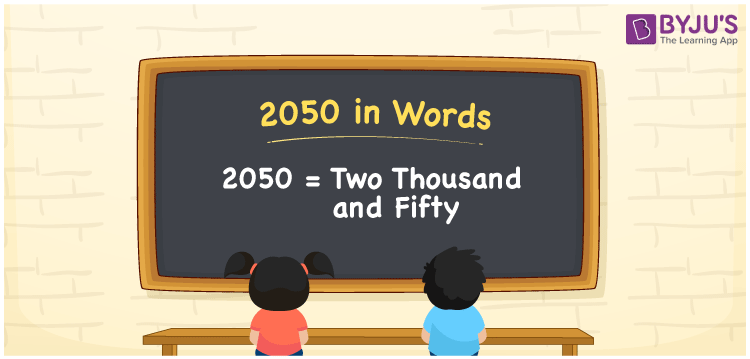# 2050 in Words

2050 in words is written as Two thousand fifty. In both the International System of Numerals and the Indian System of Numerals, 2050 is written as Two thousand fifty. The number 2050 is a Cardinal Number as it represents some quantity. For example, “the dress costs 2050 rupees”.

 2050 in words Two thousand fifty Two thousand fifty in Number 2050

## 2050 in English Words

2050 in English words is read as “Two thousand fifty.”## How to Write 2050 in Words?

To write 2050 in words, we shall use the place value chart. In the place value chart, put 2 in the thousands, 0 in the hundreds, 5 in the tens, and 0 in the ones, respectively. Let us make a place value chart to write the number 2050 in words.

 Thousands Hundreds Tens Ones 2 0 5 0

Thus, we can write the expanded form as

2 × Thousand + 0 × Hundred + 5 × Ten + 0 × One

= 2 × 1000 + 0 × 100 + 5 × 10 + 0 × 1

= 2000 + 0 + 50 + 0

= 2050

= Two thousand fifty.

2050 is a natural number which is the successor of 2049 and the predecessor of 2051.

2050 in words – Two thousand fifty

• Is 2050 an odd number? – No
• Is 2050 an even number? – Yes
• Is 2050 a perfect square number? – No
• Is 2050 a perfect cube number? – No
• Is 2050 a prime number? – No
• Is 2050 a composite number? – Yes

## Frequently Asked Questions on 2050 in Words

Q1

### How to write 2050 in words?

2050 in words is written as Two thousand fifty.
Q2

### How to write 2050 in words in the International and Indian System of Numerals?

In both, the system of numerals, 2050 in words, is written as Two thousand fifty.
Q3

### How to write 2050 in a place value chart?

In the place value chart, write 2 in the thousands, 0 in the hundreds place, 5 in the tens, and 0 in the ones, respectively.